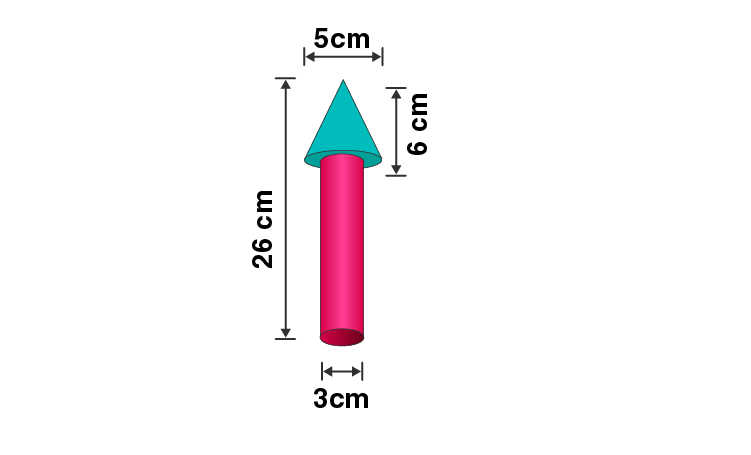Newbie

# This figure is of a wooden toy rocket, it’s in shape of a circular cone mounted on a circular cylinder. The total height of the rocket is 26 cm, while the height of the conical part is 6 cm. The base of the conical portion has a diameter of 5 cm, while the base diameter of the cylindrical portion is 3 cm. If the conical portion is to be painted green and the cylindrical portion red, find the area of the rocket painted with each of these colours. Also find the volume of the wood in the rocket.

• 0

ICSE Board Question Based on Mensuration Chapter of M.L Aggarwal for class10
This figure shows a wooden toy rocket which is in the shape of a circular cone mounted on a circular cylinder. The total height ,the height of the conical part , the base of the conical portion diameter and the base diameter of the cylindrical portion is given.
This is the Question Number 13, Exercise 17.4 of M.L Aggarwal.

Share

1.(i) Given height of the rocket = 26 cm

Height of the cone, H = 6 cm

Height of the cylinder, h = 26-6 = 20 cm

Diameter of the cone = 5 cm

Radius of the cone, R = 5/2 = 2.5 cm

Diameter of the cylinder = 3 cm

Radius of the cylinder, r = 3/2 = 1.5 cm

Slant height of cone, , l = √(H2+r2)

= √(62+2.52)

= √(36+6.25)

= √(42.25)

= 6.5 cm

Curved surface area of the cone = Rl

= 3.14×2.5×6.5

= 51.025 cm2

Base area of cone = R2

= 3.14×2.52

= 3.14×6.25

= 19.625 cm2

Curved surface area of the cylinder = 2rh

= 2×3.14×1.5×20

= 188.4 cm2

Base area of cylinder = r2

= 3.14×1.52

= 3.14×2.25

= 7.065 cm2

Total surface area of conical portion to be painted green = Curved surface area of the cone+ Base area of cone- Base area of cylinder

= 51.025 + 19.625 – 7.065

= 63.585 cm2

= 63.59 cm2

Hence the area of the rocket painted with green colour is 63.59 cm2.

Total surface area of the cylindrical portion to be painted red = Curved surface area of the cylinder + Base area of cylinder

= 188.4+7.065

= 195.465 cm2

= 195.47 cm2

Hence the area of the rocket painted with red colour is 195.47 cm2.

(ii) Volume of wood in the rocket = Volume of cone + Volume of cylinder

= (1/3)R2H + r2h

= ((R2H/3) + r2h))

= 3.14×((2.52×6/3) + 1.52×20)

= 3.14×((6.25×2) + 2.25×20)

= 3.14×(12.5+ 45)

= 3.14×57.5

= 180.55 cm3

Hence the volume of the wood in the rocket is 180.55 cm3.

• 2